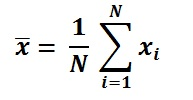## Mean Value Calculator

 Enter Inputs 5,20,40,80,100 Enter the Numbers with Comma separated(,)
 Result: No.of Inputs Mean

The Arithmetic Mean Calculator to find out Arithmetic Mean of the given data set. The symbol of mean represented as .

### Arithmetic Mean Calculator formula

To a set of data {x1, x2, ..., xn}, The arithmetic mean of it is:For example, to a data set {5,20,40,80,100}, No.of Inputs is 5, sum of it is 245, so the arithmetic mean is 245/5 = 49.

Thinkcalculator.com provides you helpful and handy calculator resources.